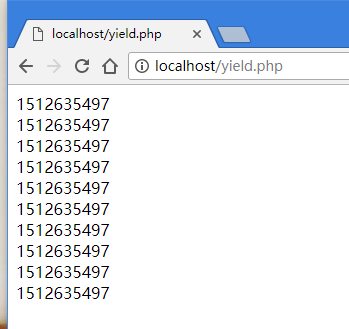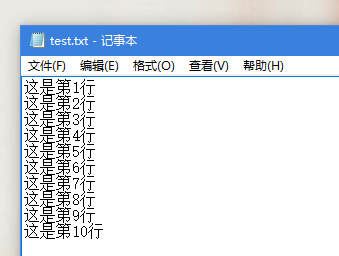# PHP性能优化利器：生成器 yield理解

#### 概念引入

``````function createRange(\$number){
\$data = [];
for(\$i=0;\$i<\$number;\$i++){
\$data[] = time();
}
return \$data;
}``````

``````\$result = createRange(10); // 这里调用上面我们创建的函数
foreach(\$result as \$value){
sleep(1);//这里停顿1秒，我们后续有用
echo \$value.'<br />';
}``````#### 创建生成器

``````function createRange(\$number){
for(\$i=0;\$i<\$number;\$i++){
yield time();
}
}``````

#### 使用生成器

``````\$result = createRange(10); // 这里调用上面我们创建的函数
foreach(\$result as \$value){
sleep(1);
echo \$value.'<br />';
}``````我们奇迹般的发现了，输出的值和第一次没有使用生成器的不一样。这里的值（时间戳）中间间隔了1秒。

#### 深入理解生成器

##### 代码剖析

``````function createRange(\$number){
for(\$i=0;\$i<\$number;\$i++){
yield time();
}
}

\$result = createRange(10); // 这里调用上面我们创建的函数
foreach(\$result as \$value){
sleep(1);
echo \$value.'<br />';
}``````

• 1 . 首先调用 createRange 函数，传入参数10，但是 for 值执行了一次然后停止了，并且告诉 foreach 第一次循环可以用的值。
• 2 . foreach 开始对 \$result 循环，进来首先 sleep(1) ，然后开始使用 for 给的一个值执行输出。
• 3.foreach 准备第二次循环，开始第二次循环之前，它向 for 循环又请求了一次。
• 4.for 循环于是又执行了一次，将生成的时间戳告诉 foreach .
• 5.foreach 拿到第二个值，并且输出。由于 foreach 中 sleep(1) ，所以， for 循环延迟了1秒生成当前时间

#### 实际开发应用

##### 读取超大文件

PHP开发很多时候都要读取大文件，比如csv文件、text文件，或者一些日志文件。这些文件如果很大，比如5个G。这时，直接一次性把所有的内容读取到内存中计算不太现实。``````<?php
{
# code...
\$handle = fopen("./test.txt", 'rb');

while (feof(\$handle)===false) {
# code...
yield fgets(\$handle);
}

fclose(\$handle);
}

foreach (readTxt() as \$key => \$value) {
# code...
echo \$value.'<br />';
}``````通过上图的输出结果我们可以看出代码完全正常。

##### 百万级别的访问量

yield生成器是php5.5之后出现的，yield提供了一种更容易的方法来实现简单的迭代对象，相比较定义类实现 Iterator 接口的方式，性能开销和复杂性大大降低。

yield生成器允许你 在 foreach 代码块中写代码来迭代一组数据而不需要在内存中创建一个数组。

``````/**
* 计算平方数列
* @param \$start
* @param \$stop
* @return Generator
*/
function squares(\$start, \$stop) {
if (\$start < \$stop) {
for (\$i = \$start; \$i <= \$stop; \$i++) {
yield \$i => \$i * \$i;
}
}
else {
for (\$i = \$start; \$i >= \$stop; \$i--) {
yield \$i => \$i * \$i; //迭代生成数组： 键=》值
}
}
}
foreach (squares(3, 15) as \$n => \$square) {
echo \$n . ‘squared is‘ . \$square . ‘<br>‘;
}

3 squared is 9
4 squared is 16
5 squared is 25
...  ``````

``````/对某一数组进行加权处理

//通常方法，如果是百万级别的访问量，这种方法会占用极大内存
function rand_weight(\$numbers)
{
\$total = 0;
foreach (\$numbers as \$number => \$weight) {
\$total += \$weight;
\$distribution[\$number] = \$total;
}
\$rand = mt_rand(0, \$total-1);

foreach (\$distribution as \$num => \$weight) {
if (\$rand < \$weight) return \$num;
}
}

//改用yield生成器
function mt_rand_weight(\$numbers) {
\$total = 0;
foreach (\$numbers as \$number => \$weight) {
\$total += \$weight;
yield \$number => \$total;
}
}

function mt_rand_generator(\$numbers)
{
\$total = array_sum(\$numbers);
\$rand = mt_rand(0, \$total -1);
foreach (mt_rand_weight(\$numbers) as \$num => \$weight) {
if (\$rand < \$weight) return \$num;
}
}``````